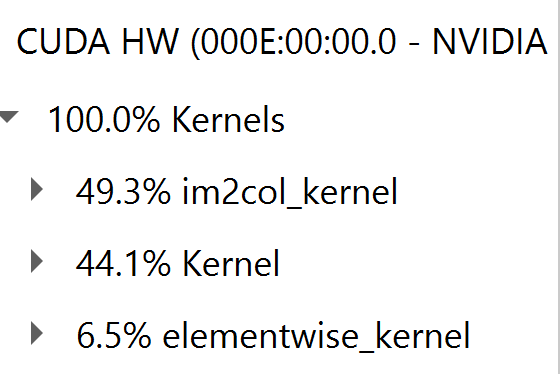# Bfloat16 has worse performance than float16 for conv2d

Hi,
I just compared the performance of my model with different parameter data types, and I found that using bfloat16 would get worse performance than float16. Is it expected or not?

For the further investigation, I used simple models to compare the performance of bfloat16 and float16.

exp1.

input = torch.randn(20, 16, 500, 1000)
m = nn.Conv2d(16, 33, 3, stride=2)
for _ in range(1000):
output = m(input)

exp2.

input = torch.randn(10000, 10000)
m = nn.Linear(10000, 20000)
for _ in range(1000):
output = m(input)

For exp1, the execution time of float16/bfloat16/float32 was 2.1/3.8/3.2 s. while for exp2, the execution time of float16/bfloat16/float32 was 20.1/19.5/33.8 s.
For conv2, the performance of bfloat16 was even was than float32. Is this expected?

By the way, all my experiments were done by A100 GPUs (80 GB) with torch 1.11.0, cuda 11.1.3, NCCL 2.8.4

In your code snippet you are not using the GPU, so I’m unsure if the code snippet is wrong or if you are measuring the CPU performance and the GPU information is irrelevant.
If you are using the device, then note that synchronizations are needed.
To enable bfloat16 support for conv layers, you would have to enable the cuDNN v8 API via `TORCH_CUDNN_V8_API_ENABLED=1` and would need a build with this API enabled via `USE_EXPERIMENTAL_CUDNN_V8_API=1` (the current nightly binaries should already build with it).

Actually, my original code was,

exp2.

input = torch.randn(10000, 10000).to(device=_gpu_device, dtype=_type)
m = nn.Linear(10000, 20000).to(device=_gpu_device, dtype=_type)
torch.cuda.synchronize()
import time
t = time.time()
for _ in range(100):
output = m(input)
torch.cuda.synchronize()
time_elapse = time.time() - t
print(f"time_elapse = {time_elapse}")

Exp1 is written in the same way. Since I wanted to make my exp easy to be read, I just simplified it.
Thanks for you comments, I will tried environment variables you mentioned.

@ptrblck , I have tried to set the environment variables but found no gain for bfloat16 performance.

On the other hand, I profiled my experiment using Nvidia Nsight Systems. The diagram showed that different kernels were applied for float16 and bfloat16. Did I run model with bfloat16 correctly?
bfloat16 profiling result